# Lecture 16 - Shortest paths I - BFS and Djikstra

Date Pre-lecture slides Post-lecture scribbles Lecture recording
October 19 2023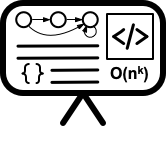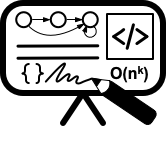## Notes

Breadth First Search (BFS) is a graph traversal algorithm that visits all the vertices of a graph in breadth-first order, i.e., it visits all the vertices at distance 1 from the starting vertex, then all the vertices at distance 2, and so on.

BFS is implemented using a queue data structure, which stores the vertices in the order they are visited. The algorithm starts at a specified vertex, marks it as visited, and adds it to the queue. Then it dequeues the first vertex from the queue, visits all its unvisited neighbors, marks them as visited, and adds them to the queue. This process continues until the queue is empty.In the above graph, if we start at Node with value 2, the BFS would result in two traversals: (2, 3, 0, 1) or (2, 0, 3, 1).

### Time Complexity

 The time complexity of BFS is O( V + E ), where V is the number of vertices and E is the number of edges in the graph. This is because each vertex and edge is visited at most once.

## Dijkstra’s Algorithm for Shortest Path

Dijkstra’s algorithm is a graph traversal algorithm that is used to find the shortest path between a starting vertex and all other vertices in a weighted graph. The algorithm maintains a set of visited vertices and a set of unvisited vertices, and it iteratively selects the unvisited vertex with the smallest distance from the starting vertex and visits all its neighboring vertices.

The algorithm works as follows:

1. Initialize the distance of the starting vertex to 0, and the distance of all other vertices to infinity. Mark all vertices as unvisited.
2. Select the unvisited vertex with the smallest distance from the starting vertex, and mark it as visited.
3. For each neighboring vertex that is still unvisited, calculate its tentative distance from the starting vertex by adding the weight of the edge between the two vertices to the distance of the current vertex. If this tentative distance is smaller than the current distance of the neighboring vertex, update its distance to the tentative distance.
4. Repeat steps 2 and 3 until all vertices have been visited, or until the destination vertex (if specified) has been visited.

At the end of the algorithm, the shortest distance from the starting vertex to all other vertices in the graph will have been calculated.

### Example: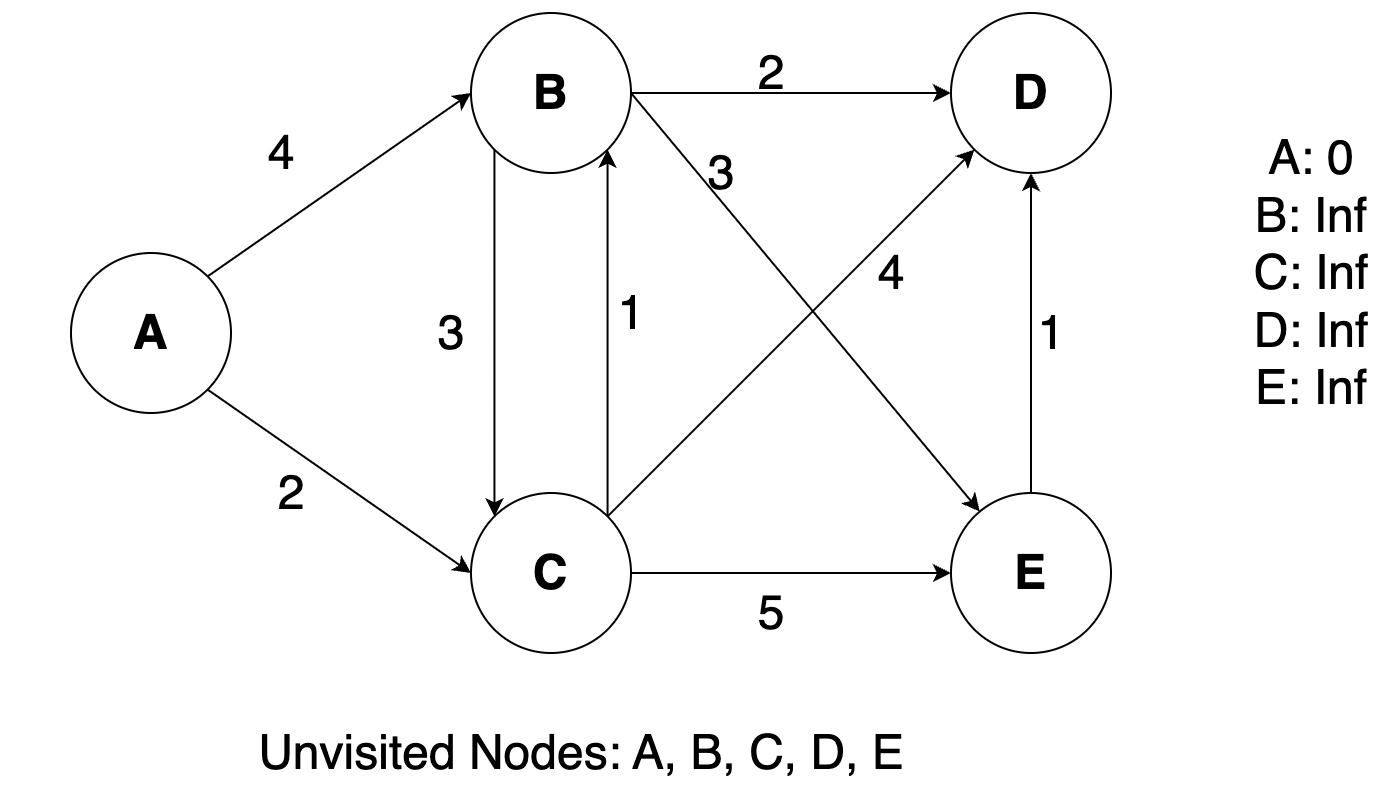We will find the min distance from A to all other nodes in the graph. Initially the distance will be mapped as Infinity (inf) for all nodes other than A and we will also maintain an unvisited Array at the bottom. First we will mark A as visited and then update the distance maps based on the nodes that A has edges to.We see that C is the next unvisited node with shortest distance, so we will now mark C as visited and update the distance map.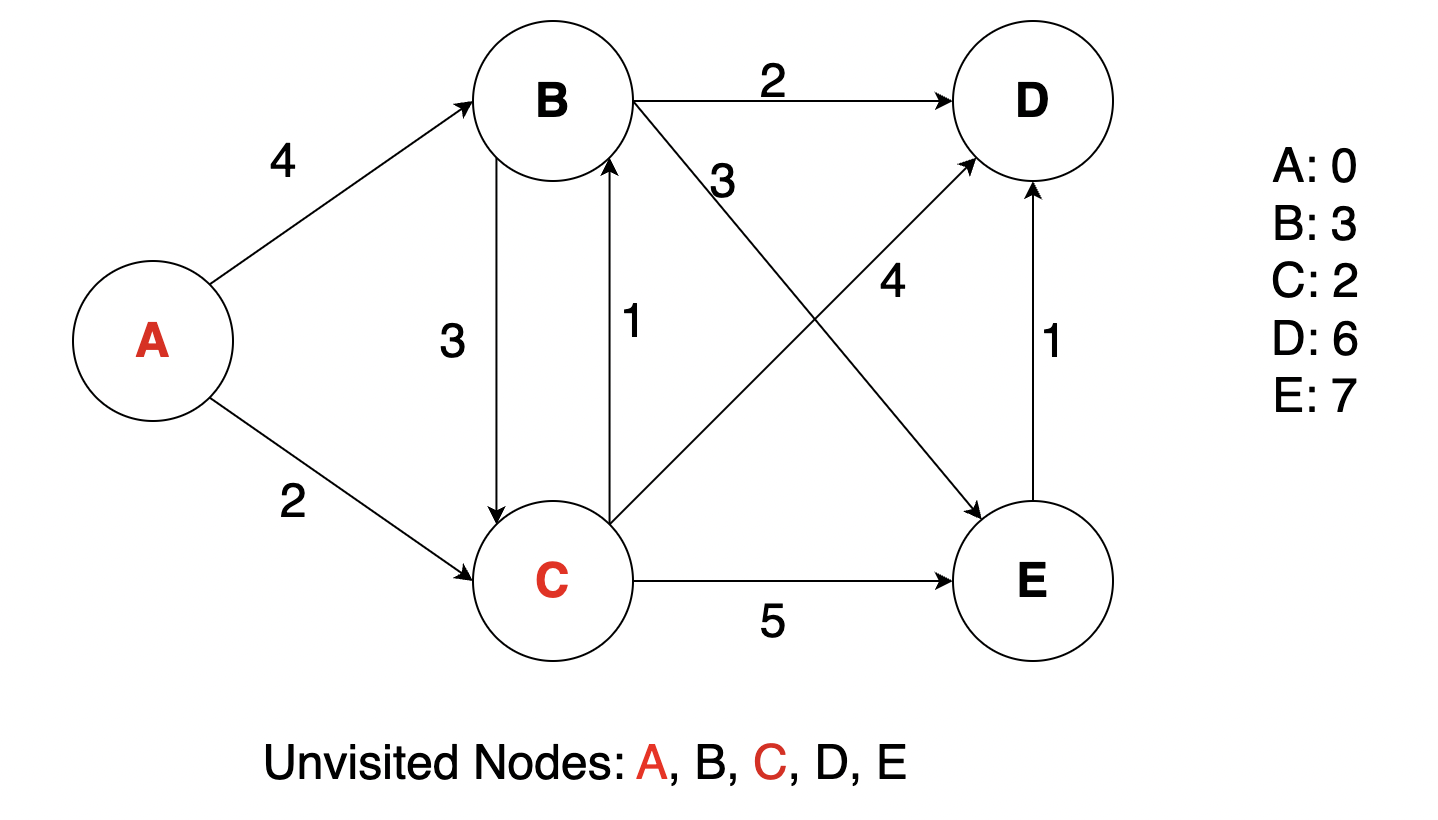Next we do similar steps with B, D, E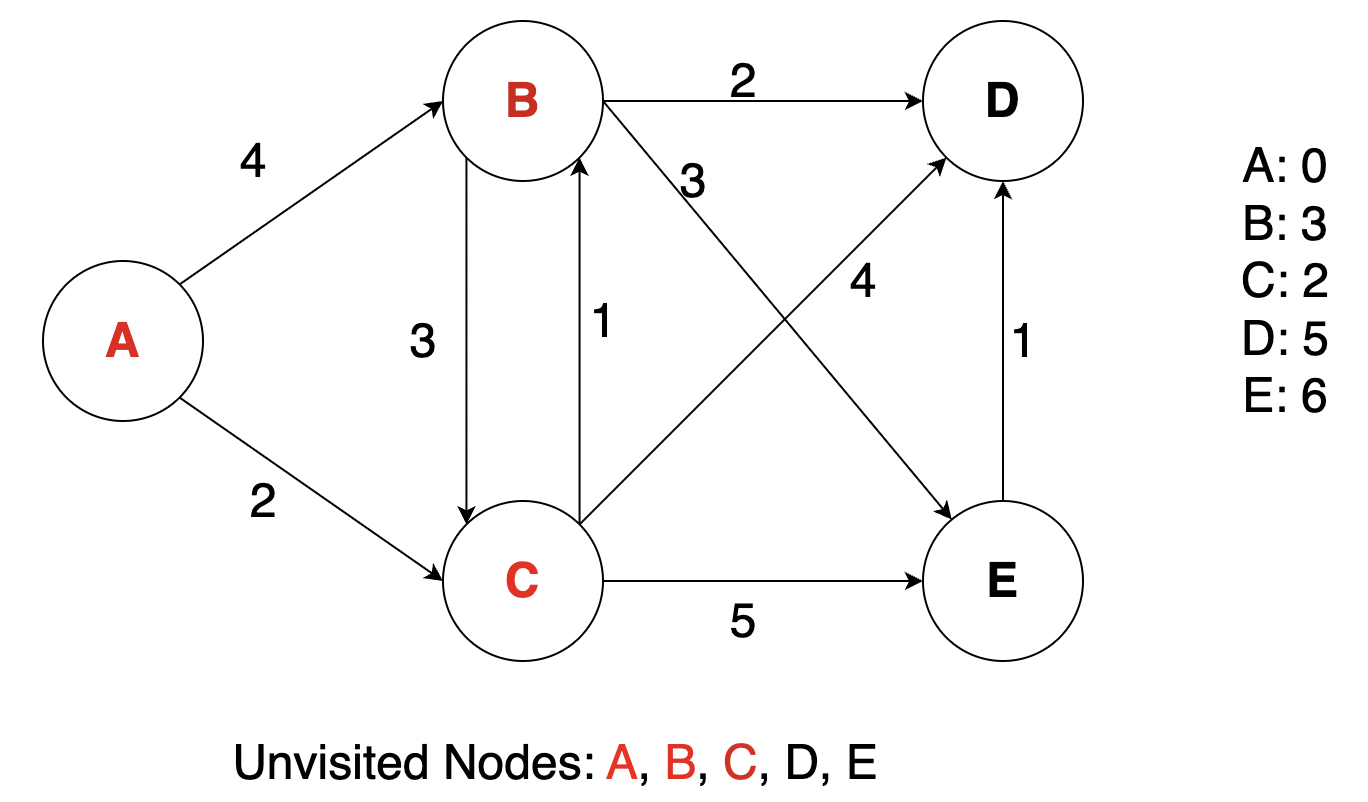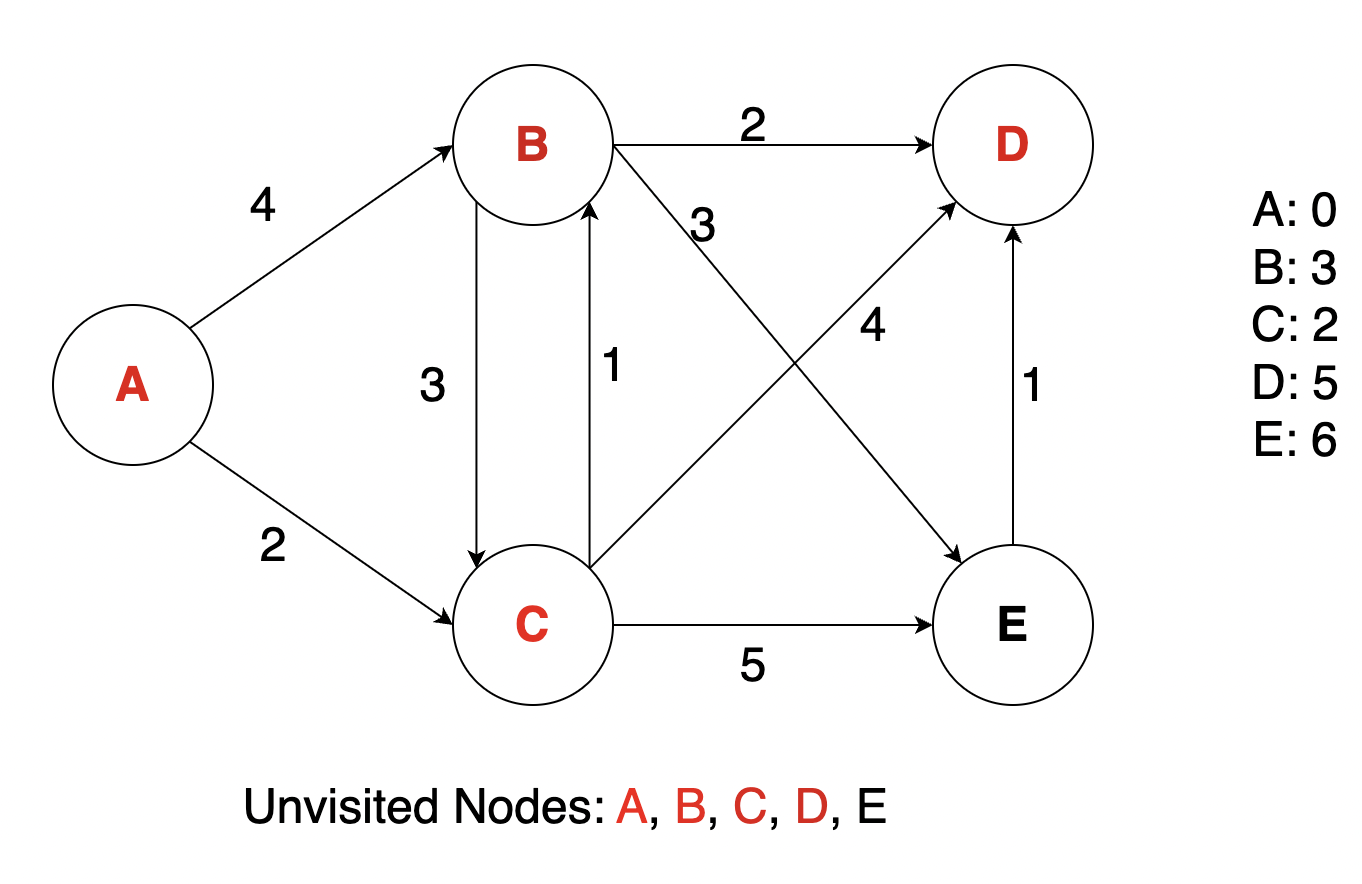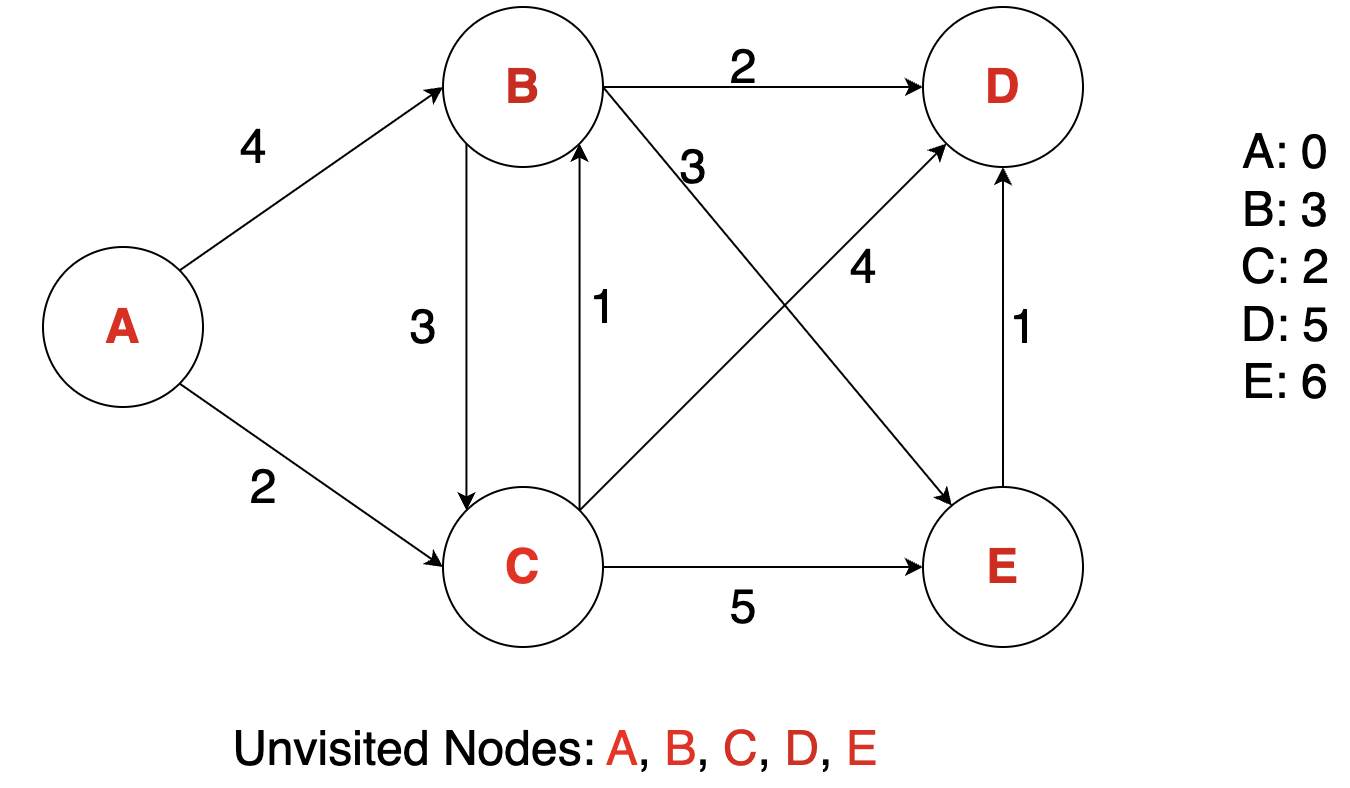### Time Complexity

 The time complexity of Dijkstra’s algorithm is O( E + V log V ), where V is the number of vertices and E is the number of edges in the graph. This is because the algorithm performs a total of E relaxation steps (i.e., updating distances to neighboring vertices) and V extract-min operations (i.e., selecting the unvisited vertex with the smallest distance).

## Dijkstra’s Algorithm with Priority Queue

Dijkstra’s algorithm can be implemented using a priority queue data structure to efficiently select the unvisited vertex with the smallest distance from the starting vertex.

The algorithm works as follows:

1. Initialize the distance of the starting vertex to 0, and the distance of all other vertices to infinity. Insert all vertices into the priority queue.
2. While the priority queue is not empty, remove the vertex with the smallest distance from the starting vertex (the root of the priority queue).
3. If the removed vertex is the destination vertex (if specified), terminate the algorithm and return the shortest distance.
4. For each neighboring vertex of the removed vertex that is still in the priority queue, calculate its tentative distance from the starting vertex by adding the weight of the edge between the two vertices to the distance of the removed vertex. If this tentative distance is smaller than the current distance of the neighboring vertex, update its distance to the tentative distance and update its position in the priority queue accordingly.
5. Repeat steps 2-4 until the destination vertex has been visited, or until the priority queue is empty.

### Time Complexity

 The time complexity of Dijkstra’s algorithm with a priority queue is O(( E + V ) log V ), where V is the number of vertices and E is the number of edges in the graph. This is because the algorithm performs a total of E relaxation steps (i.e., updating distances to neighboring vertices) and V extract-min operations (i.e., selecting the unvisited vertex with the smallest distance) using a priority queue with O(log V ) time complexity.

• Textbooks
• Erickson, Jeff. Algorithms
• Skiena, Steven. The Algorithms Design Manual
• Chapter 7.6 - Breadth-First Search
• Chapter 8.3 - Shortest Path
• Sedgewick, Robert and Wayne, Kevin. Algorithms (Forth Edition)
• Chapter 4.1 - Breadth-First Search
• Chapter 4.4 - Shortest Paths
• Cormen, Thomas, et al. Algorithms (Forth Edition)
• Chapter 20.2 - Breadth-First Search
• Chapter 22.1 - The Bellman-Ford Algorithm
• Sariel’s Lecture 17